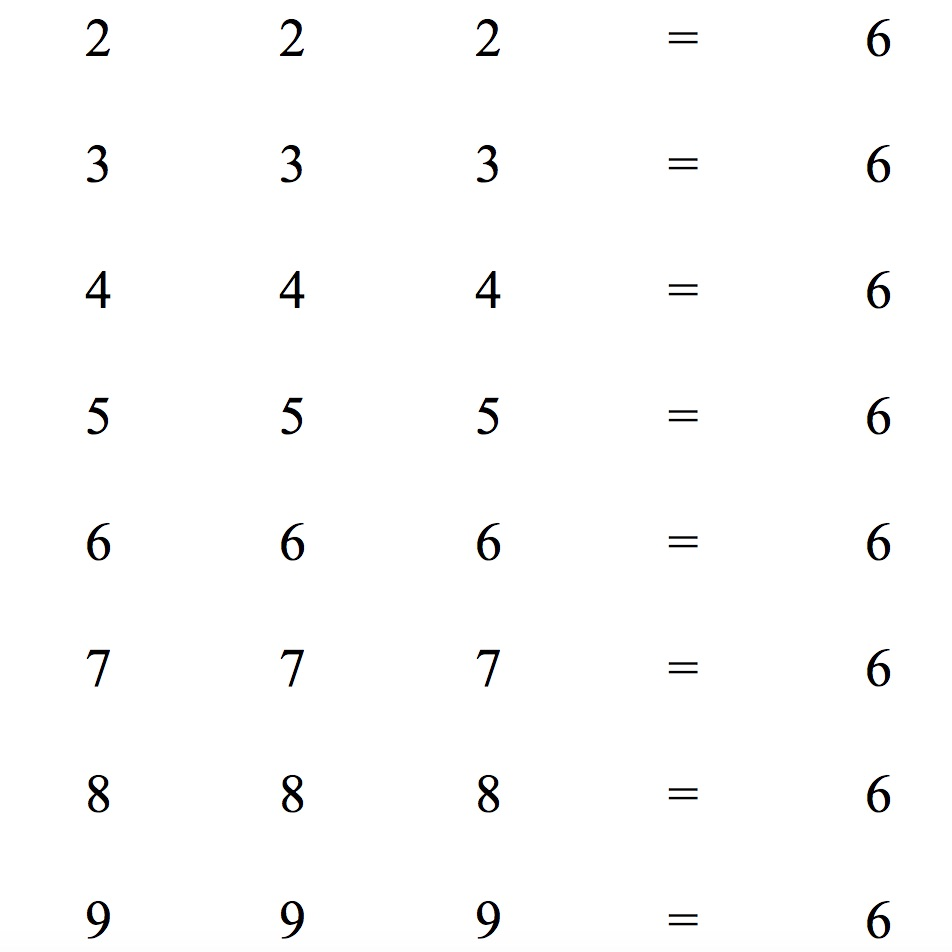Insert signs and symbols to make each line a true equation.

Hint: You can use square roots and cube roots.

As an extension you could investigate the factorial sign on your calculator and use factorials to answer the same questions for 0  0  0 = 6 and 1  1  1 = 6. For example 1! = 1 and 4!= 24.

See the Notes for Teachers

Tagged with: